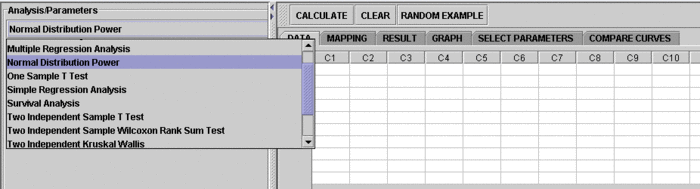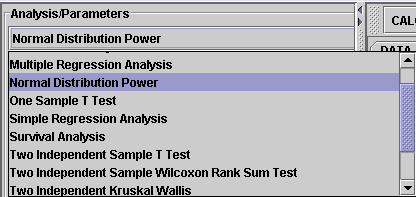# SOCR EduMaterials AnalysisActivities NormalPower

## SOCR Analysis Example on Power Analysis for Normal Distribution

### This SOCR Activity demonstrates the utilization of the SOCR Analyses package for statistical Computing. In particular, it shows how to use Power Analysis Tool for Normal Distribution and how to read the results.

• As you start the SOCR Analyses Applet, click on "Normal Distribution Power" from the combo box in the left panel. Here's what the screen should look like.There are FOUR things you can do with the SOCR Normal Power Analysis Activity:

• This activity lets the user input his own sample size, means of the null and alternative hypothesis, standard deviation, and significance level. The power will be computed, and graph of power will be provided. Normal curves of the data, as well as the distributions of the estimated means, will be displayed.
• This activity is similar to the one above, but the computer generates a set of data and part of the input is computed using the data, such as the sample size, the mean pf the null and the standard deviation. The user than needs to specify the mean of the alternative and significance level, for power analysis.
• This one works the "opposite" of the above two. Power is given by the user and sample size is computed.
• This simple activity takes user-specified mean, standard deviation and significance level, with any given number "x", and computes for the z-score of x.